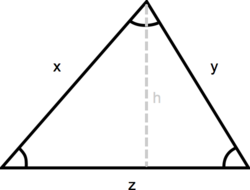## ASVAB Math Knowledge Practice Test 153002

Question 1 of 5
###### Triangle Geometry

A triangle is a three-sided polygon. It has three interior angles that add up to 180° (a + b + c = 180°). An exterior angle of a triangle is equal to the sum of the two interior angles that are opposite (d = b + c). The perimeter of a triangle is equal to the sum of the lengths of its three sides, the height of a triangle is equal to the length from the base to the opposite vertex (angle) and the area equals one-half triangle base x height: a = ½ base x height.If the base of this triangle is 1 and the height is 7, what is the area?

 3$$\frac{1}{2}$$ 49$$\frac{1}{2}$$ 112$$\frac{1}{2}$$ 32$$\frac{1}{2}$$